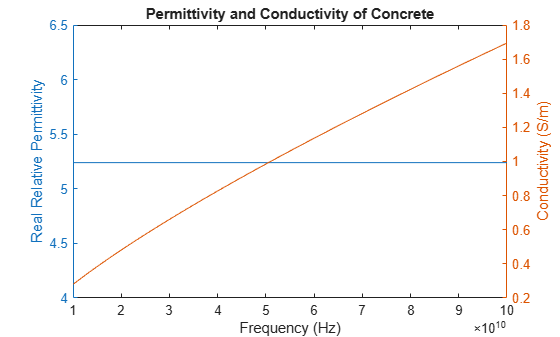# buildingMaterialPermittivity

Permittivity and conductivity of building materials

Since R2020a

## Syntax

``[epsilon,sigma,complexepsilon] = buildingMaterialPermittivity(material,fc)``

## Description

example

````[epsilon,sigma,complexepsilon] = buildingMaterialPermittivity(material,fc)` calculates the relative permittivity, conductivity, and complex relative permittivity for the specified material at the specified frequency. The methods and equations modeled in the `buildingMaterialPermittivity` function are presented in Recommendation ITU-R P.2040 .```

## Examples

collapse all

Calculate relative permittivity and conductivity at 9 GHz for various building materials as defined by textual classifications in ITU-R P.2040, Table 3.

```material = ["vacuum";"concrete";"brick";"plasterboard";"wood"; ... "glass";"ceiling-board";"chipboard";"floorboard";"metal"]; fc = repmat(9e9,size(material)); % Frequency in Hz [permittivity,conductivity] = ... arrayfun(@(x,y)buildingMaterialPermittivity(x,y),material,fc);```

Display the results in a table.

```varNames = ["Material";"Permittivity";"Conductivity"]; table(material,permittivity,conductivity,'VariableNames',varNames)```
```ans=10×3 table Material Permittivity Conductivity _______________ ____________ ____________ "vacuum" 1 0 "concrete" 5.31 0.19305 "brick" 3.75 0.038 "plasterboard" 2.94 0.054914 "wood" 1.99 0.049528 "glass" 6.27 0.059075 "ceiling-board" 1.5 0.0064437 "chipboard" 2.58 0.12044 "floorboard" 3.66 0.085726 "metal" 1 1e+07 ```

Calculate the relative permittivity and conductivity for concrete at frequencies specified.

```fc = ((1:1:10)*10e9); % Frequency in Hz [permittivity,conductivity] = ... arrayfun(@(y)buildingMaterialPermittivity("concrete",y),fc);```

Plot the relative permittivity and conductivity of concrete across the range of frequencies.

```figure yyaxis left plot(fc,permittivity) ylabel('Relative Permittivity') yyaxis right plot(fc,conductivity) ylabel('Conductivity (S/m)') xlabel('Frequency (Hz)') title('Permittivity and Conductivity of Concrete')```## Input Arguments

collapse all

Building material, specified as vector of strings, or an equivalent character vector or cell array of character vectors including one or more of these options:

 `"vacuum"` `"glass"` `"very-dry-ground"` `"concrete"` `"ceiling-board"` `"medium-dry-ground"` `"brick"` `"floorboard"` `"wet-ground"` `"plasterboard"` `"chipboard"` `"wood"` `"metal"`

Example: `["vacuum" "brick"]`

Data Types: `char` | `string`

Carrier frequency in Hz, specified as a positive scalar.

Note

`fc` must be in the range [1e6, 10e6] when the `material` is `"very-dry-ground"`, `"medium-dry-ground"` or `"wet-ground"`.

Data Types: `double`

## Output Arguments

collapse all

Relative permittivity of the building material, returned as a nonnegative scalar or row vector. The output dimension of `epsilon` matches that of the input argument `material`. For more information about the computation for the relative permittivity, see ITU Building Materials.

Conductivity, in Siemens/m, of the building material, returned as a nonnegative scalar or row vector. The output dimension of `sigma` matches that of the input argument `material`. For more information about the computation for the conductivity, see ITU Building Materials.

Complex relative permittivity of the building material, returned as a complex scalar or row vector of complex values.

The output dimension of `complexepsilon` matches that of the input argument `material`. For more information about the computation for the complex relative permittivity, see ITU Building Materials.

collapse all

### ITU Building Materials

Section 3 of ITU-R P.2040-1  presents methods, equations, and values used to calculate real relative permittivity, conductivity, and complex relative permittivity at carrier frequencies up to 100 GHz for common building materials.

The `buildingMaterialPermittivity` function uses equations from ITU-R P.2040-1 to compute these values.

• The real part of the relative permittivity is calculated as

`epsilon` = afb.

The computation of `epsilon` is based on equation (58). f is the frequency in GHz. Values for a and b are specified in Table 3 from ITU-R P.2040-1.

• The conductivity in Siemens/m is calculated as

`sigma` = cfd.

The computation of `sigma` is based on equation (59). f is the frequency in GHz. Values for c and d are specified in Table 3 from ITU-R P.2040-1.

• The complex permittivity is calculated as

`complexepsilon` = `epsilon` – 1i `sigma` / (2πfcε0).

The computation of `complexepsilon` is based on Equations (59) and (9b). fc is the carrier frequency in GHz. ε0 = 8.854187817e-12 Farads/m, where ε0 is the electric constant for the permittivity of free space.

For cases where the value of b or d is zero, the corresponding value of `epsilon` or `sigma` is a or c, respectively and independent of frequency.

The contents of Table 3 from ITU-R P.2040-1 are repeated in this table. The values a, b, c, and d are used to calculate relative permittivity and conductivity. Except as noted for the three ground types, the frequency ranges given in the table are not hard limits but are indicative of the measurements used to derive the models. The `buildingMaterialPermittivity` function interpolates or extrapolates relative permittivity and conductivity values for frequencies that fall outside of the noted limits. To compute relative permittivity and conductivity for different types of ground as a function carrier frequencies up to 1000 GHz, see the `earthSurfacePermittivity` function.

Material ClassReal Part of Relative PermittivityConductivity (S/m)Frequency Range (GHz)
abcd

Vacuum (~ air)

1

0

0

0

[0.001, 100]

Concrete

5.31

0

0.0326

0.8095

[1, 100]

Brick

3.75

0

0.038

0

[1, 10]

Plasterboard

2.94

0

0.0116

0.7076

[1, 100]

Wood

1.99

0

0.0047

1.0718

[0.001, 100]

Glass

6.27

0

0.0043

1.1925

[0.1, 100]

Ceiling board

1.50

0

0.0005

1.1634

[1, 100]

Chipboard

2.58

0

0.0217

0.78

[1, 100]

Floorboard

3.66

0

0.0044

1.3515

[50, 100]

Metal

1

0

107

0

[1, 100]

Very dry ground

3

0

0.00015

2.52

[1, 10] only(a)

Medium dry ground

15

– 0.1

0.035

1.63

[1, 10] only(a)

Wet ground

30

– 0.4

0.15

1.30

[1, 10] only(a)

Note (a): For the three ground types (very dry, medium dry, and wet), the noted frequency limits cannot be exceeded.

 International Telecommunications Union Radiocommunication Sector. Effects of building materials and structures on radiowave propagation above about 100MHz. Recommendation P.2040-1. ITU-R, approved July 29, 2015. https://www.itu.int/rec/R-REC-P.2040/en.